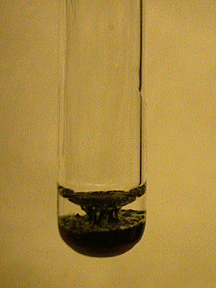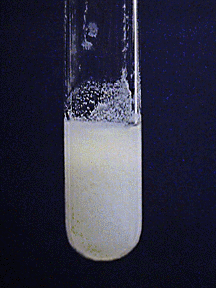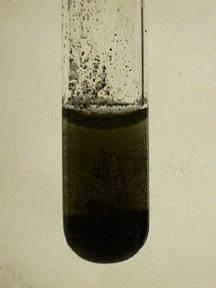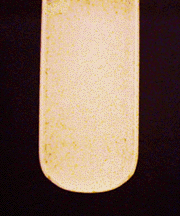# Characteristic Reactions of Mercury Ions (Hg²⁺ and Hg₂²⁺)

$$\newcommand{\vecs}{\overset { \rightharpoonup} {\mathbf{#1}} }$$ $$\newcommand{\vecd}{\overset{-\!-\!\rightharpoonup}{\vphantom{a}\smash {#1}}}$$$$\newcommand{\id}{\mathrm{id}}$$ $$\newcommand{\Span}{\mathrm{span}}$$ $$\newcommand{\kernel}{\mathrm{null}\,}$$ $$\newcommand{\range}{\mathrm{range}\,}$$ $$\newcommand{\RealPart}{\mathrm{Re}}$$ $$\newcommand{\ImaginaryPart}{\mathrm{Im}}$$ $$\newcommand{\Argument}{\mathrm{Arg}}$$ $$\newcommand{\norm}{\| #1 \|}$$ $$\newcommand{\inner}{\langle #1, #2 \rangle}$$ $$\newcommand{\Span}{\mathrm{span}}$$ $$\newcommand{\id}{\mathrm{id}}$$ $$\newcommand{\Span}{\mathrm{span}}$$ $$\newcommand{\kernel}{\mathrm{null}\,}$$ $$\newcommand{\range}{\mathrm{range}\,}$$ $$\newcommand{\RealPart}{\mathrm{Re}}$$ $$\newcommand{\ImaginaryPart}{\mathrm{Im}}$$ $$\newcommand{\Argument}{\mathrm{Arg}}$$ $$\newcommand{\norm}{\| #1 \|}$$ $$\newcommand{\inner}{\langle #1, #2 \rangle}$$ $$\newcommand{\Span}{\mathrm{span}}$$

• Most common oxidation states: +1, +2
• M.P. -38.87º
• B.P. 356.57º
• Density 13.546 g/cm3
• Characteristics: Mercury is one of the few liquid elements. It dissolves in oxidizing acids, producing either $$\ce{Hg^{2+}}$$ or $$\ce{Hg_2^{2+}}$$, depending on which reagent is in excess. The metal is also soluble in aqua regia ( a mixture of hydrochloric and nitric acids) to form $$\ce{HgCl4^{2-}}$$.

## Mercury(I) Ion: Hg₂²⁺

Mercury(I) compounds often undergo disproportionation, producing black metallic mercury and mercury(II) compounds.

### Chloride Ion

Soluble chlorides, including hydrochloric acid, precipitate white mercury(I) chloride, also known as calomel:

$\ce{Hg_2^{2+}(aq) + 2Cl^{-}(aq) <=> Hg2Cl2(s)}$Aqueous ammonia reacts with $$\ce{Hg2Cl2}$$ to produce metallic mercury (black) and mercury(II) amidochloride (white), a disproportionation reaction:

$\ce{Hg2Cl2(s) + 2NH3(aq) -> Hg(l) + HgNH2Cl(s) + NH4^{+}(aq) + Cl^{-}(aq)}$### Aqueous Ammonia

Aqueous ammonia produces a mixture of a white basic amido salt and metallic mercury:

$\ce{2Hg_2^{2+}(aq) + 4NH3(aq) + NO3^{-}(aq) + H2O(l) -> 2Hg(l) + Hg2ONH2NO3(s) + 3NH4^{+}(aq)}$The precipitate is not soluble in excess aqueous ammonia.

### Sodium Hydroxide

Black finely divided mercury metal and yellow mercury(II) oxide ($$\ce{HgO}$$) are precipitated by $$\ce{NaOH}$$:

$\ce{Hg_2^{2+}(aq) + 2OH^{-}(aq) -> Hg(l) + HgO(aq) + H2O(l)}$### Reducing Agents

Reducing agents, such as $$\ce{Sn^{2+}}$$ and $$\ce{Fe^{2+}}$$, reduce mercury(I) to the metal:

$\ce{Hg_2^{2+}(aq) + 2Fe^{2+}(aq) -> 2Hg(l) + 2Fe^{3+}(aq) }$Consult an activity series or a table of reduction potentials for other possible reducing agents.

### No Reaction

$$\ce{SO4^{2-}}$$ (unless solutions are concentrated; solubility of mercury(I) sulfate is 0.06 g per 100 mL of water at 25oC)

## Mercury(II) Ion: Hg²⁺

Characteristic reactions of $$\ce{Hg^{2+}}$$

### Chloride Ion

No reaction is visible, but Hg(II) will be present as $$\ce{[HgCl4]^{2-}}$$.

### Aqueous Ammonia

Aqueous ammonia produces white amido salts whose composition depends on the mercury(II) salt present in the solution:

$\ce{HgCl2(aq) + 2NH3(aq) <=> HgNH2Cl(s) + 2NH4^{+}(aq) + Cl^{-}(aq)}$These salts are not soluble in excess aqueous ammonia, but do dissolve in acids:

$\ce{HgNH2Cl(s) + 2H^{+}(aq) + Cl^{-}(aq) <=> HgCl2(aq) + NH4^{+}(aq) }$### Sodium Hydroxide

A yellow precipitate of $$\ce{HgO}$$ is produced by $$\ce{NaOH}$$:

$\ce{Hg^{2+}(aq) + 2OH^{-}(aq) -> HgO(s) + H2O(l)}$

$\ce{HgCl2(s) + 2OH^{-}(aq) -> HgO(s) + H2O(l) + 2Cl^{-}(aq)}$The mercury(II) oxide precipitate is insoluble in excess hydroxide but is soluble in acids:

$\ce{HgO(s) + 2H^{+}(aq) <=> Hg^{2+}(aq) + H2O(l)}$### Hydrogen Sulfide

Hydrogen sulfide precipitates black mercury(II) sulfide, the least soluble of all sulfide salts.

$\ce{Hg^{2+}(aq) + H2S(aq) <=> HgS(s) + 2H^{+}(aq)}$

$\ce{[HgCl4]^{2-}(aq) + H2S(aq) <=> HgS(s) + 2H^{+}(aq) + 4Cl^{-}(aq)}$Mercury(II) sulfide is insoluble in 6 M $$\ce{HNO3}$$ or 12 M $$\ce{HCl}$$, even if heated. However, it is soluble in aqua regia (3:1 HCl:HNO3) and in hot dilute $$\ce{NaOH}$$ containing excess sulfide.

$\ce{3HgS(s) + 12Cl^{-}(aq) + 2NO3^{-}(aq) + 8H^{+}(aq) -> 3[HgCl4]^{2-}(aq) + 2NO(g) + 3S(s) + 4H2O(l)}$

$\ce{HgS(s) + S2^{-}(aq) <=> [HgS2]^{2-}(aq)}$

### Tin(II) Chloride

Tin(II) chloride reduces $$\ce{Hg(II)}$$ to $$\ce{Hg(I)}$$ or to metallic $$\ce{Hg}$$, giving a white or gray precipitate:

$\ce{2[HgCl4]^{2-}(aq) + [SnCl4]^{2-}(aq) <=> Hg2Cl2(s) + [SnCl6]^{2-}(aq) + 4Cl^{-}(aq)}$### No Reaction

$$\ce{SO4^{2-}}$$ (may precipitate as a mixed sulfate-oxide - a basic sulfate - $$\ce{HgSO4 \cdot 2HgO}$$)

Characteristic Reactions of Mercury Ions (Hg²⁺ and Hg₂²⁺) is shared under a not declared license and was authored, remixed, and/or curated by James P. Birk.